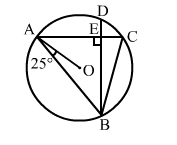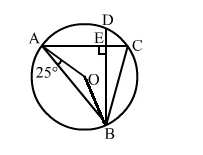# In the adjoining figure, chords AC and BD of a circle with centre O,

Question:

In the adjoining figure, chords AC and BD of a circle with centre O, intersect at right angles at E. If OAB = 25°, calculate ∠EBC.Solution:

OA = OB (Radii of a circle)
Thus, OBA = OAB = 25°
Join OB.Now in ΔOAB, we have:
OAB + OBA + AOB = 180° (Angle sum property of a triangle)
">25° + 25° + AOB = 180°
">50° + AOB = 180°
">AOB = (180° – 50°) = 130°

We know that the angle subtended by an arc of a circle at the centre is double the angle subtended by the arc at any point on the circumference.
i.e., AOB = 2ACB

$\Rightarrow \angle A C B=\frac{1}{2} \angle A O B=\left(\frac{1}{2} \times 130^{\circ}\right)=65^{\circ}$

Here,ACB = ECB
∴ ECB = 65°   ...(i)

Considering the right angled ΔBEC, we have:
EBC + BEC + ECB = 180°     (Angle sum property of a triangle)
">EBC + 90° + 65° = 180°    [From(i)]
">EBC = (180° – 155°) = 25°
Hence, EBC = 25°